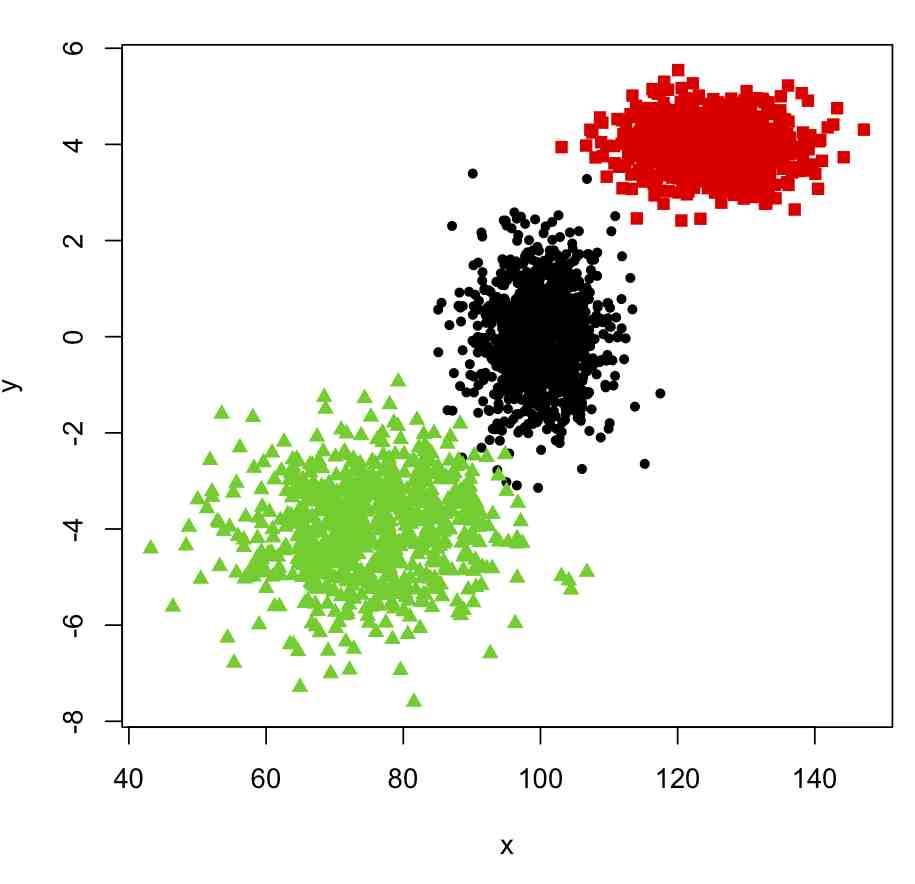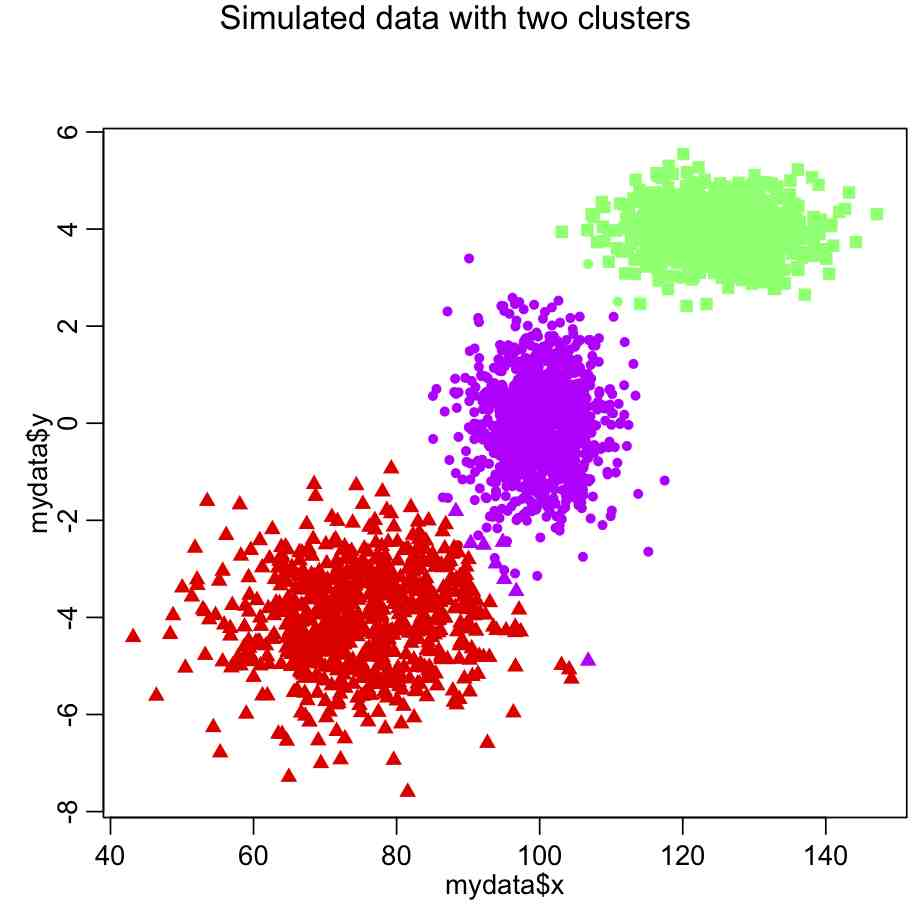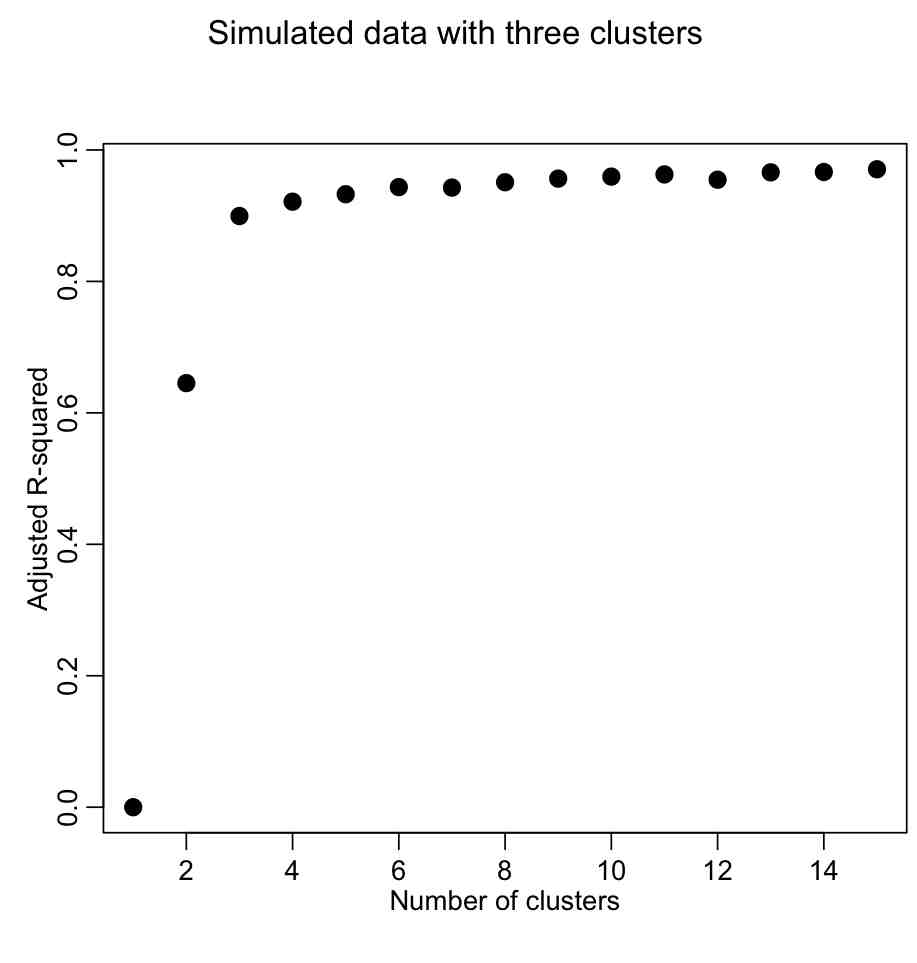# AML610 Fall 2013 lecture series

Introduction

In this course students will be introduced to statistical modelling methods such as linear regression, factor regression, and time series analysis.  All modelling and data analysis will be performed in the R statistical programming language.  The course meets on Thursdays from 12:00-2:45 pm in PSA 546.  The course syllabus can be found here.

The course will be structured in a series of modules covering various topics.  Some modules may take more than one lecture to cover.  Homework will be occasionally assigned throughout the course, usually after completion of a module.

The final project for the course will account for 50% of the grade, and is required to be based on a statistical analysis of one or more data sets from this page and in conjunction with discussion with myself and perhaps other faculty to determine if the topic of the analysis is novel.  Students are encouraged to work together on the project in groups of up to three, but the contributions of each student in the group to the project must be clearly defined.  The final project write-up will be due approximately two weeks before the end of classes (date to be announced).  Oral presentations of the projects will take place in class during the last week of classes.

There is no one textbook that covers the material in this course. Since students in this course have varying backgrounds in statistics, I strongly recommend that you go to the library and take a look at the statistical texts there, and find one or two that cover linear regression and/or time series analysis that you think are at your level. For recommended texts that I think are good, Statistical Data Analysis by Cowan is a good general text for various statistical methods.  A standard textbook for linear regression is Applied Linear Statistical Models by Kutner et al.  Two good textbooks that cover time series analysis is Time Series Analysis with Applications in R by Cryer, and Time Series Analysis and its Applications with R Examples by Shumway.  The material covered in these texts is much more expansive in scope than the material covered in this course (because they cover material that would form the basis of at least three or four different courses).  All of these texts are available off of libgen.info, but note that copyright infringement is a crime… one must never do such a terrible thing.

Visits: 904

# The basics of the R statistical progamming language

[After you have read through this module, and have downloaded and worked through the provided R examples, you should be proficient enough in R to be able to download and run other R scripts that will be provided in other posts on this site. You should understand the basics of good programming practices (in any language, not just R). You will also have learned how to read data in a file into a table in R, and produce a plot.]

Contents:

Why use R for modelling?

I have programmed in many different computing and scripting languages, but the ones I most commonly use on a day to day basis are C++, Fortran, Perl, and R (with some Python, Java, and Ruby on the side).  In particular, I use R every day because it is not only a programming language, but also has graphics and a very large suite of statistical tools. Connecting models to data is a process that requires statistical tools, and R provides those tools, plus a lot more.

Unlike SAS, Stata, SPSS, and Matlab, R is free and open source (it is hard to beat a package that is more comprehensive than pretty much any other product out there and is free!).

Visits: 2038
| Tagged

# The difference between mathematical and statistical modelling (plus some more basics of R)

[In this module, we will discuss the difference between mathematical and statistical modelling, using pandemic influenza as an example.  Example R code that solves the differential equations of a compartmental SIR model with seasonal transmission (ie; a mathematical model) is presented.  Also provided are an example of how to download add-on library packages in R, plus more examples of reading data sets into R, and aggregating the data sets by some quantity (in this case, a time series of influenza data in Geneva in 1918, aggregated by weekday).

Delving into how to write the R code to solve systems of ODE’s related to a compartmental mathematical model is perhaps slightly off the topic of a statistical modelling course, but worthwhile to examine; as mathematical and computational modellers, usually your aim in performing statistical analyses will be to uncover potential relationships that can be included in a mathematical model to make that model better describe the underlying dynamics of the system]

Visits: 11527

# Review of Probability Distributions, Basic Statistics, and Hypothesis Testing

[In this module, students will learn about probability distributions important to statistical modelling, focussing primarily on probability distributions that underlie the stochasticity in time series data.

In addition, in this course we will be learning how to formulate figure-of-merit statistics that can help to answer research questions like “Is quantity A significantly greater/less than quantity B?”, or “Does quantity X appear to be significantly related to quantity Y?”.  As we are about to discuss, statistics that can be used to answer these types of questions do so using the underlying probability distribution to the statistic.  Every statistic used for hypothesis testing has an underlying probability distribution.]

Visits: 7142

# Hypothesis testing of sample means (flowchart)

On this page we give the flow chart for testing means of independent samples. For instance, the set of temperature measurements over a 10 year period for all days in July is pretty independent of the set of temperature measurements over a 10 year period for all days in January.  An example of non-independent samples is the measurement of cancer tumor size in 100 patients before and after some cancer treatment; the final tumor size will of course be somewhat (or a lot) correlated to the tumor size at the beginning of treatment.

Visits: 1490

# Least Squares linear regression

In this module, students will become familiar with least squares linear regression methods. Note that before proceeding with any regression analysis, it is important to first perform initial data exploration,and numerical summaries of the variables like the mean, standard deviations, maxima and minima, and correlations between variables.  In this way, you can determine if there are any unexpected “quirks” or problems with the data (and, more often than not, there are).

Visits: 3010

Visits: 1374

# Correlations, Partial Correlations, and Confounding Variables

In this post we discuss the calculation of the correlation coefficient between two variables, X and Y, and the partial correlation coefficient which controls for the effect of a potential confounding variable, Z

Visits: 8663

# Hypothesis testing of count data (flowchart)

This page describes how to determine if count data are statistically consistent with some value. Count data are data counted in bins of some time span, for instance the number of influenza cases per day, or the number of murders per year.

Visits: 2744

# Exploratory Data Analysis: examples

Exploratory data analysis essentially is the process of getting to know your data by making plots and perhaps doing some simple statistical hypothesis tests.  Getting to know your data is important before starting the process of regression analysis or any kind of more advanced hypothesis testing, because, more often than not, real data will have “issues” that complicate statistical analyses.

Visits: 1427

# Hypothesis testing example problems

Example problems involving hypothesis testing

Visits: 1572

# K-means clustering

Sometimes we may want to determine if there are apparent “clusters” in our data (perhaps temporal/geo-spatial clusters, for instance).  Clustering analyses form an important aspect of large scale data-mining.

K-means clustering partitions n observations into k clusters, in which all the points are assigned to the cluster with the nearest mean.

If you decide to cluster the data in to k clusters, essentially what the algorithm does is to minimize the within-cluster sum of squared distances of each point to the center of the cluster (does this sound familiar?  It is similar to Least Squares).

The algorithm starts by randomly picking k points to be the cluster centers.  Then each of the remaining points is assigned to the cluster nearest to it by Euclidian distance.  In the next step, the mean centroid of each of the clusters is calculated from the points assigned to the cluster.  Then the algorithm re-assigns all points to the their nearest cluster centroid.  Then the centroid of each cluster is updated with the new point assignments. And then the points are re-assigned to clusters.  And the cluster centroids re-calculated… and so on and so on until you reach a step where none of the points switch clusters. Visually, the algorithm looks like this:How many clusters are in the data?

You likely want to explore how many distinct clusters appear to be in the data. To do this, you can examine how much of the variance in the data is described by our “model” (which in this case is the clustered data).  Just like for a linear least squares statistical model, we can thus calculate an adjusted R-squared from the total variance in the data and the sum of the within group variance.  There is also a way to calculate the AIC for a kmeans model.

To see how to implement kmeans clustering in R, examine the following code that generates simulated data in 3 clusters:

```require("sfsmisc")
set.seed(375523)
n1 = 1000
x1 = rnorm(n1,100,5)
y1 = rnorm(n1,0,1)
n2 = 750
x2 = rnorm(n2,125,7)
y2 = rnorm(n2,4,0.5)
n3 = 1500
x3 = rnorm(n2,75,10)
y3 = rnorm(n2,-4,1)

x = c(x1,x2,x3)
y = c(y1,y2,y3)
true_clus = c(rep(1,n1),rep(2,n2),rep(3,n3))
pch_clus = c(rep(20,n1),rep(15,n2),rep(17,n3))
mydata = data.frame(x,y)
plot(x,y,col=true_clus,pch=pch_clus)```

This produces the following plot:Now, to cluster these into nclus clusters, we can use the kmeans() function in R, like this

```mydata_for_clustering=mydata
n = nrow(mydata_for_clustering)
nclus = 3
myclus = kmeans(mydata_for_clustering,centers=nclus)
print(names(myclus))

###################################################################
# plot the data, colored by the clusters
###################################################################
mult.fig(1,main="Simulated data with two clusters")
scol = rainbow(nclus,end=0.8) # select colors from the rainbow
plot(mydata\$x,mydata\$y,col=scol[myclus\$cluster],pch=pch_clus)```

Do you see something wrong here?

The problem is that the x dimension has a much larger range than the y dimension, and so in the calculation of the Euclidean distance it carries far too much weight. The solution is to normalize the space such that all dimensions have the same dimensionality. Try this:

```mydata_for_clustering=mydata
mydata_for_clustering\$x = (x-mean(x))/sd(x)
mydata_for_clustering\$y = (y-mean(y))/sd(y)
nclus = 3

myclus = kmeans(mydata_for_clustering,centers=nclus)
print(names(myclus))

###################################################################
# plot the data, colored by the clusters
###################################################################
mult.fig(1,main="Simulated data with two clusters")
scol = rainbow(nclus,end=0.8) # select colors from the rainbow
plot(mydata\$x,mydata\$y,col=scol[myclus\$cluster],pch=pch_clus)```

which yields the following:In most cases, however, the number of clusters won’t be so obvious.  In which case, we would need to use a figure of merit statistic like adjusted R^2 or (even better) AIC to determine which number of clusters appears to best describe the data.

To calculate the r-squared for a variety of cluster sizes, do this (after making sure your data are normalized!):

```####################################################################
# the data frame mydata_for_clustering is ncol=2 dimensional
# and we wish to determine the minimum number of clusters that leads
# to a more-or-less minimum total within group sum of squares
####################################################################
kmax = 15 # the maximum number of clusters we will examine; you can change this
totwss = rep(0,kmax) # will be filled with total sum of within group sum squares
kmfit = list() # create and empty list
for (i in 1:kmax){
kclus = kmeans(mydata_for_clustering,centers=i,iter.max=20)
totwss[i] = kclus\$tot.withinss
kmfit[[i]] = kclus
}

####################################################################
# and plot it
####################################################################
rsq = 1-(totwss*(n-1))/(totwss*(n-seq(1,kmax)))

mult.fig(1,main="Simulated data with three clusters")

Producing the following plot:We want to describe as much of the R^2 with the fewest number of clusters.  The usual method is to look for the “elbow” in the plot, and that is traditionally done visually.  However, we could try to construct a figure-of-merit statistic that identifies the elbow.  In the AML610 course, the students and the professor developed a quantitative method to formulate a statistic to identify the elbow point.  We call this statistic the COMINGS-elbow statistic (after the first initials of the students who came up with the algorithm); the statistic looks for the (k,aic) point that has the closest euclidean distance to (1,min(aic)).  However, we noted that the performance of this statistic in identifying the elbow point was improved if we first normalized the k and aic vectors such that they lay between 0 to 1.  Thus, the elbow point is identified by the (k’,aic’) point that is closest to (0,0).

```####################################################################
# try to locate the "elbow" point
####################################################################
vrsq = (rsq-min(rsq))/(max(rsq)-min(rsq))
k = seq(1,length(vrsq))
vk = (k - min(k))/(max(k)-min(k))
dsq = (vk)^2 + (vrsq-1)^2

cat("The apparent number of clusters is: ",nclus,"\n")
points(nclus,rsq[nclus],col=2,pch=20,cex=2)```

This figure-of-merit for elbow location doesn’t seem to work optimally. Can we think of a better one?

The AIC can be calculated with the following function:

```kmeansAIC = function(fit){

m = ncol(fit\$centers)
n = length(fit\$cluster)
k = nrow(fit\$centers)
D = fit\$tot.withinss
return(D + 2*m*k)
}

aic=sapply(kmfit,kmeansAIC)
mult.fig(1,main="Simulated data with two clusters")
plot(seq(1,kmax),aic,xlab="Number of clusters",ylab="AIC",pch=20,cex=2)```

Again, we are looking for the elbow point in the plot.

```####################################################################
# look for the elbow point
####################################################################
v = -diff(aic)
nv = length(v)
fom = v[1:(nv-1)]/v[2:nv]
nclus = which.max(fom)+1
cat("The apparent number of clusters is: ",nclus,"\n")
points(nclus,aic[nclus],col=2,pch=20,cex=2)```

With the AIC, our elbow locator FoM seems to work better (in this case, anyway).

In the file kmeans_example.R, I show the location of terrorist attacks in Afghanistan between 2009 to 2011 from the Global Terrorism Database.   The data are in afghanistan_terror_attacks_2009_to_2011.txt

Visits: 12319

# AML 610 Fall module V: Time series analysis

[In this module, students will become familiar with time series analysis methods, including lagged regression methods, Fourier spectral analysis, harmonic linear regression, and Lomb-Scargle spectral analysis]

Visits: 4697